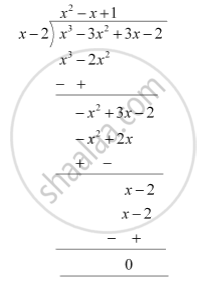# On dividing x^3 – 3x^2 + x + 2 by a polynomial g(x), the quotient and remainder were x – 2 and –2x + 4, respectively. Find g(x) - Mathematics

On dividing x3 – 3x2 + x + 2 by a polynomial g(x), the quotient and remainder were x – 2 and –2x + 4, respectively. Find g(x)

#### Solution

p(x) = x3-3x2+x+2 (Dividend)

g(x) = ? (Divisor)

Quotient = (x - 2)

Remainder = (-2x + 4)

Dividend = Divisor × Quotient + Remainder

x3 - 3x2 + x + 2 = g(x) x (x - 2) + (-2x + 4)

x3 - 3x2 + x + 2 +2x - 4 = g(x)(x - 2)

x3 - 3x2 + 3x - 2 = g(x)(x - 2)

g(x) is the quotient when we divide (x3 -3x2 + 3x - 2) by (x - 2)∴ g(x) = (x^2 - x + 1)

Concept: Division Algorithm for Polynomials
Is there an error in this question or solution?
Chapter 2: Polynomials - Exercise 2.3 [Page 36]

#### APPEARS IN

NCERT Class 10 Maths
Chapter 2 Polynomials
Exercise 2.3 | Q 4 | Page 36

Share